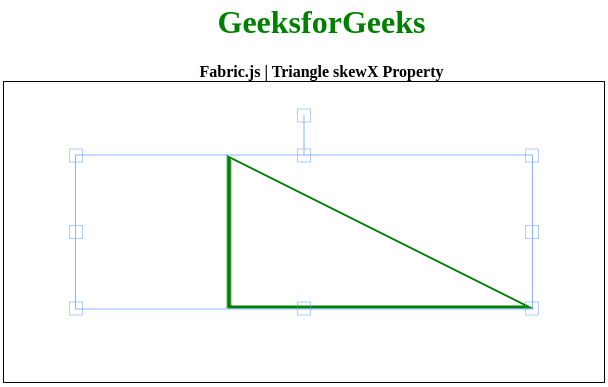Related Articles

# Fabric.js | Triangle skewX Property

• Last Updated : 27 May, 2020

In this article, we are going to see how to set the horizontal skew of a canvas triangle using FabricJS. The canvas triangle means the triangle is movable and can be stretched according to requirement. Further, the triangle can be customized when it comes to initial stroke color, height, width, fill color, or stroke width.

To make it possible we are going to use a JavaScript library called FabricJS. After importing the library, we will create a canvas block in the body tag that will contain the triangle. After this, we will initialize instances of Canvas and Triangle provided by FabricJS and set horizontal skew of the triangle using skewX property and render the Triangle on the Canvas as given in the example below.

Syntax:

```fabric.Triangle({
width: number,
height: number,
skewX: number
});```

Parameters: This function accepts three parameters as mentioned above and described below:

• width: It specifies the width of triangle.
• height: It specifies the height of triangle.
• skewX: It specifies the horizontal skew.

Example: This example uses FabricJS to set horizontal skew of a canvas triangle.

 ` `` `` ` ` ``    `` ``        ``Fabric.js | Triangle skewX Property``    `` ``     ` `    ````    `` `` `` ` ` ``    ``
``      ``

``       ``GeeksforGeeks``      ``

``      ````       ``Fabric.js | Triangle skewX Property``      ````    ``
``    `` ``    `` ``     ` `    `` `` `` ` ``

Output:My Personal Notes arrow_drop_up